## Filtration of Liquid from a Point Source in a Soil Mass.

1 Moscow State University of Civil Engineering, Yaroslavskoe shosse, 26, Moscow, 129337, Russia.

*Corresponding Author:Vladimir Andreev, Moscow State University of Civil Engineering, Yaroslavskoe shosse, 26, Moscow, 129337, Russia, Tel: +7 (499) 183-83-65; Fax: +7 (499) 183-44-38; E-mail: asv@mgsu.ru

Citation: Vladimir Andreev, Anatoliy Avershyev (2023) Filtration of Liquid from a Point Source in a Soil Mass. SciEnvironm 6: 174.

Received: March 22, 2023; Accepted: April 07, 2023; Published: April 12, 2023.

## Abstract

The rupture of water and sewer pipes leads to numerous accidents (subsidence, swelling, etc.). This is especially characteristic for clay, loess, peat and other soils. We consider the stress state of clay array at different models on fluid propagation from a fracture site. Accordingly, the problems are solved in cylindrical and spherical coordinates. The problem is solved by the methods of stationary and non-stationary moisture elasticity. The feature of the calculation is the accounting of the inhomogeneity of the clay during moistening.

## Introduction

One of the topical problems of soil mechanics is the problem of the stressed state of the soil massif when the underground pipeline is broken. The flowing moisture liquefies the adjacent soil; there are forced (humid) deformations that are constrained due to ground rejection. Well-known numerous accidents on the roads due to erosion of the ground, destruction of the road surface, etc. The problem of moisture elasticity, which combines the problem of moisture conductivity (moisture transfer) and the problem of mechanics, allows determining the stressed state of the soil massif when the underground pipeline is ruptured. The formulation of the problem also consists of two independent sections: the formulation of the problem of moisture elasticity and the formulation of the problem of mechanics, in this case the theory of elasticity. First, the model of the problem of elasticity theory will be considered, since in the problem of moisture conductivity the model partially depends on the mechanical model.

## Formulation of the problem

Mechanical model

Figure 1: Various calculation schemes for solving the problem of stress distribution near an underground cylindrical cavity.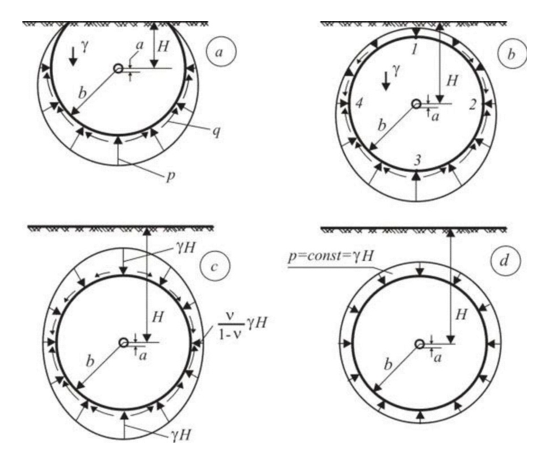Figure 1 shows the calculation schemes used in solving problems on the stressed state of a rock massif with a hole. Scheme a) corresponds to the close location of the hole to the surface of the earth and the solution can be obtained only by a numerical method. The remaining schemes differ in the ratio of the depth of the cavity H to hole radius a, and the ratio of the fictitiously excised array radius b to the radius a. Under condition H>>b>> a, we obtain a scheme d) corresponding to an axisymmetric problem (for a cylindrical hole) or a centrally symmetric problem (for a spherical hole). The solutions given below correspond to this scheme, that is, the problems are one-dimensional.

Inhomogeneity of Soils

For some soils (clay, loess, peat, etc.), humidification is characterized by a strong swelling, which leads to softening of the material. This leads to a significant change in the modulus of deformation of soil E. Figures 2, 3 show the experimental dependences of E on the relative humidity, where WS the humidity of the saturated soil [1, 2].

A mathematical dependence E(w) is necessary for calculations. The most suitable was the power function (in Figure 3, the approximating dependence is shown):(1)

Accounting inhomogeneity in the calculations leads to significant complications, since instead of differential equations with constant coefficients (at E = const.), It is necessary to solve equations with variable coefficients.

Figure 2: Dependence of the deformation modulus of soil on its humidity: 1 - loam and clay;2 - sandy loam.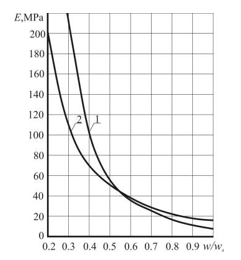Figure 3: Dependence of the deformation modulus of clay on relative humidity: 1 - experimental dependence; 2 - approximating function .Statement of the problems of moisture transfer

Before proceeding with the formulation of the problem, let us consider the models of the propagation of a liquid from the place where a pipe is broken. Figure 4 shows two models considered below. In the model shown in Figure 4,a, it is assumed that there is a gap between the soil and the pipe, and moisture first moves along the pipe, and then spreads to the ground in such a way that its front is a cylindrical surface. The second model (Figure 4, b) corresponds to the propagation of moisture with a spherical front of its motion.

Figure 4: Models moisture distribution in soil at pipe rupture: a) the distribution of moisture with a cylindrical front; b) distribution of moisture with a spherical front.

(a)                                                      (b)Below, we consider the problems of moisture transfer in cylindrical and spherical coordinates in accordance with the mechanical model described in 2.1 (Figure 1,d) and the models shown in Figure 4. In order to obtain solutions for any material that does not take into account the physical characteristics of the material, we will use dimensionless coordinates: where CW- coefficient of moisture conductivity.

The second Fick law taking into account the replacement of (1) takes the form: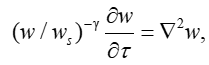(2)

Where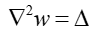– the Laplace operator, which in polar coordinates, taking into account the axial symmetry,(3)

and in spherical coordinates with allowance for the central symmetry has the form: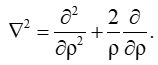(4)

The boundary conditions of the first kind for a hollow cylinder or sphere have the form: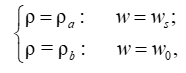(5)

where,The initial condition in nonstationary problems has the form: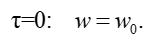(6)

Thus, the problems are reduced to the solution of partial differential equations (2) with boundary and initial conditions (5), (6). For the stationary regime both in cylindrical and spherical coordinates there is an analytical solution . To solve equation (2) in the case of nonstationary regime, the method of separation of variables  is used and the solution is obtained in series with respect to Bessel functions . Details of these solutions are given in [7, 8].

## Results and discussion

Stationary problem

As an example, we present the results of calculations for the case of the cylindrical front of fluid motion (Figure 4.1). Figure 5, 6 show graphs of dependencies stresses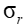and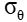obtained for the clay massif with the following initial data: a = 0,25cm; b = 2,5m; H = 10m; Ws =0.363; Cw =2.4.107  m/c2 ;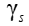=29.95 kN/m3;n =0.4.

Let's pay attention to the diagram.In the zone of tension (dangerous for soils), the stress in the inhomogeneous material is greater than in the homogeneous material, which emphasizes the importance of taking into account the inhomogeneity of the soils under moistening.

Figure 5: Diagrams of stresses––Inhomogeneous material (E= E (r)). - - - - Homogeneous material (E = const).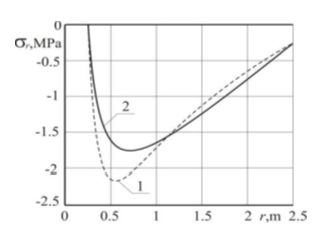Figure 6: Diagrams of stresses–– Inhomogeneous material (E= E (r)); - - - Homogeneous material (E = const).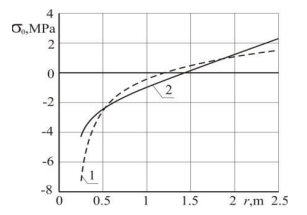Nonstationary problems

Axisymmetrically problem

Taking into account the relatively slow spread of the liquid into the soil, the problem is solved in a quasistatic formulation. Taking into account the complexity of the resolving equation, the solution is sought numerically. As was indicated above, the solution is a series of Bessel functions. These series do not converge well, and in order to obtain astable result, the calculation was carried out taking into account the 100 terms of the series. Since the circumferential stresses are the most dangerous from the point of view of strength, we shall confine ourselves to the calculation results for these stresses. Figure 7 shows the stress diagrams for different instants of time.

Figure 7: Distribution circumferential stresses along the radius at different times: a) homogeneous material. b) inhomogeneous material.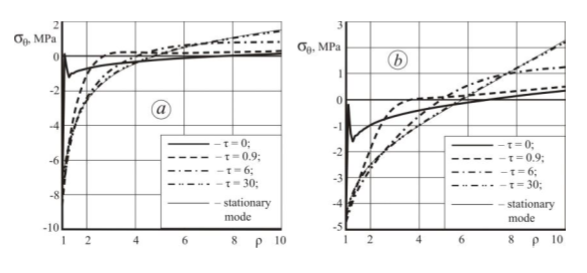The jump on the diagram corresponding to   is due to an instantaneous change in the soil moisture during the rupture of the pipe, ie, there is a singular point here, and even an increase in the number of terms in the series does not make it possible to smooth this dependence.

Based on the obtained results, two conclusions can be drawn:

1 - taking into account the inhomogeneity of the soil during swelling shows that the compressive stresses are greater in the vicinity of the tube rupture site than in the calculations for a homogeneous material, and at the periphery of the array stresses are tensile and also larger in the inhomogeneous material. Since the growth of compressive stresses does not cause danger, their growth is not principled in calculations for strength. However, the growth of tensile stresses is a danger to soils.

2 – At the instant of time   the diagram approaches the stationary curve shown in Figure 6. It follows that this time corresponds to the output of the process to the steady state.

Central symmetric problem

The solution of this problem is similar to the solution given in 3.2.1. In deriving the resolving equations, instead of the expression for the Laplace operator (3) we used the formula (4). As in the previous case, the solution was obtained by a numerical method using 100 series members. Figure 8 shows the stress diagrams   for different instants of time.

Figure 8: Distribution circumferential stresses along the radius at different times: a) homogeneous material; b) inhomogeneous material.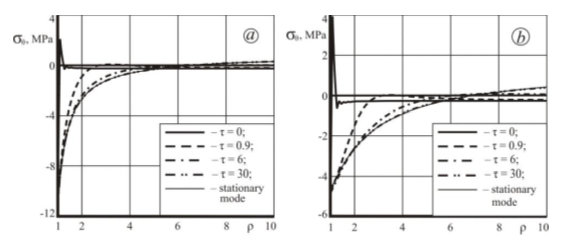Comparing this Figure with Figure 7, it can be noted that both conclusions made in 3.2.1 are valid for this case. The difference lies in the fact that when the liquid is distributed along spherical surfaces, the tensile stresses are less than in the case of the cylindrical front of the fluid. Because we probably do not know how the liquid is spreading out of the hole in the tube, it would be logical to average the results obtained for the two models shown in Figure 4.

## Conclusion

Calculations of various bodies, taking into account the inhomogeneity caused by the influence of external fields (temperature, radiation, humidity, etc.) make it possible to significantly clarify the stress-strain state of these bodies. Numerous studies, including the author [9 -11 etc.], have shown that the direction "Mechanics of inhomogeneous bodies" is topical and requires further development.

## References

1. V A Davydov (1979) Features of research and design of highways in the permafrost regions. (Omsk, Omsk PI).
2. Industry Road Norms 218.046-01. (Russia, 2000) Design of non-rigid road clothes.
3. V I Andreev (2002) Some problems and methods in mechanics of inhomogeneous bodies. (Publ. House ASV, Moscow).
4. V I Andreev, A S Avershyev (2013) Adv. Mat. Res. TTP, 671-674, 571.
5. AV Lykov (1967) Theory of heat conductivity. Moscow: Higher School.
6. E. Kamke (2003) Handbook of ordinary differential equations. (St. Petersburg, "Lan")
7. V I Andreev, A S Avershyev (2013) Trans. Int. Conf. on Fl. Str. Int., WITpress, 123.
8. V I Andreev, A S Avershyev (2014) Adv. Mat. Res. TTP, 838-841 254.
9. V I Andreev (2007) Herald of the Department of Building Sciences of the RAASN. 1. 48.
10. V I Andreev (2015) L S Polyakova, Bulletin of NR MGSU. 11 38.
11. V I Andreev (2013) Applied Mechanics and Materials. 353-354 1267.